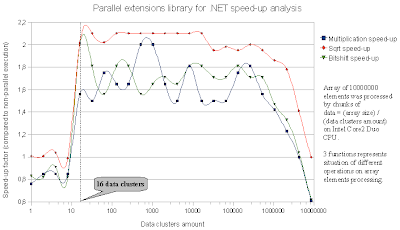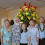## Sunday, March 30, 2008

### Parallel extensions for .NET and multi-core cpu

This time we look a bit at parallel extensions library for .NET. This library intended for speeding-up work for multi-core cpu. If you want to speed-up program in this way -you need:
• of course multi-core machine
• parallel extensions library for .NET. You can get it there
• Also .NET Framework 3.5
• And at last- you need to optimize code to be especially suitable for multi-cores.
This issue will be mentioned later.

This library was tested on Intel Core2 Duo machine with the help of C#.
Testing procedure:

• There was created array of 10000000 elements.
• This array then was initialized in sequential and parallel ways.
And perfomance measurements was done.
• When measuring perfomance, several factors was changed to look for impact on parallel execution speed:
a) Data clusters amount (array was partitioned into different number of clusters)
b) Different mathematical operations on array elements was used.

C# code which did all measurement comparisions between sequential and parallel executions is here:

`using System;class Program{    static int ProcessVariable(int i)    {        int res;        res =            //i * i        (int)Math.Sqrt(i)            //i >> 1        ;        return res;    }    static void InitArrayParallel(int[] num, int clusters)    {        int clustcount = num.Length / clusters;        Parallel.For(1, clusters + 1, k =>        {            int ixlow = (k - 1) * clustcount;            int ixhigh = ixlow + clustcount;            for (int i = ixlow; i < ixhigh; i++)            { num[i] = ProcessVariable(i); }        });    }    static void InitArraySerial(int[] num)    {        for (int i = 1; i < num.Length; i++)        { num[i] = ProcessVariable(i); }    }    static void Main(string[] args)    {        int limit = 10000000;        int[] num = new int[limit];        double ds = 0.0;        double dp = 0.0;        double speed = 0.0;        int avg_count = 6;        DateTime t1, t2;        for (int c = 1; c <= limit; c *= 2)        {            ds = 0.0;            dp = 0.0;            for (int i = 1; i <= avg_count; i++)            {                t1 = DateTime.Now;                InitArraySerial(num);                t2 = DateTime.Now;                ds += ((TimeSpan)(t2 - t1)).TotalMilliseconds;                t1 = DateTime.Now;                InitArrayParallel(num, c);                t2 = DateTime.Now;                dp += ((TimeSpan)(t2 - t1)).TotalMilliseconds;            }            speed = ds / dp;            Console.WriteLine("Data clusters {1}; Speed-up factor {0:F2}", speed, c);        }        Console.ReadLine();    }}`

Results is interesting:There results is displayed as XY graph of speed-up factor dependance on data clusters amount.
Clusters amount scale is logarithmic.

Several conclusions that can be made from this graph (and this test):

• As it was supposed to be, maximal speed-up factor is about 2
(because machine was with 2 cores)
• speed-up heavily depends on data partitions amount.
• It seems there are a lower number of data clusters -> 16, when workload starts being nicelly distributed between cores and speed-up very sharply increases. Or else - if data partitions amount is smaller then 16,- seems there are no speed-up at all, and no use of 2 cores :-)
• Speed-up may depend on operations used on array elements. For example- from graph can be seen that parallel execution speeds-up more square root operation than bitshift, or multiplication. It seems somehow Sqrt work can be distributed between cores more friendly.
• Last- there exists also upper limit of data clusters amount, on which speed-up is still noticable. So users should not divide data into very big amount of chunks also, because in that way cores are not capable to interoperate efficently. Also higher limit may be dependent on actual operations on array elements.

So all in all - its good that we got this library for .NET. It simplifies a lot writing efficent algorithm for several cores. But.. as we saw- we need to remember that its not enough to have this library for multi-cores enabled code. We need to optimized it for that- Splitting jobs between cores is a different world of programming.

#### 1 comment:

1.Thanks Investagor, Jon, Rob, and other participants.

I'm someone who uses functional programming as a means, but not an end. That goes for Parallel Extension too.

My main interest is in graphics algoritms. So I'm interested in how coding for new CPU-multi-core using F#-functional & parallel ext. architecture compares to thinking about coding for new GPU's?

Where is the best division of algorithm labor?

Comment will be posted after comment moderation.